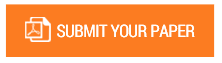The Effect of Discovery Learning Model on Students Learning Outcome at SMA Muhammadiyah 09

Authors : Rahmi Nazliah, Irmayanti, Hasmi Syahputra Harahap, Risma Delima Harahap, Zunaidy Abdullah Siregar, dan Arman Harahap

Volume/Issue : Volume 5 - 2020, Issue 3 - March

Scribd : https://bit.ly/39ED8y5

This research aims to determine the effect of dicovery learning on students learning outcomes Muhammadiyah 09 high school students. The research method used quasi-experiments with 2 classes sample which determined using cluster random sampling techniques. Science class XI1 which was consisted of 38 students taught with the control class and science class XI2 which was consisted of 35 students taught with the problem solving learning model, the instrument used in this research is the testing of student learning outcomes. Data analysis techniques used Covariat Analysis with the SPSS 22.0 for Windows program. The results of the research showed that there was an influence on the learning model. 8,050. The research post-test data in the control class taught with conventional models shows an average post-test value of 2509 with a standard deviation of 11.190. While the experimental class students who were taught with the Discovery Learning model before treatment were also pre-tested and obtained a total score of 1975 with a standard deviation of 9,672. The number of experimental post-test scores was 2905 with a standard deviation of 4.678. Based on the acquisition of the average value of the post-test on this group of students, after being given different treatment between the experimental class and the control class there are significant differences in the value of learning outcomes. This is also proved by testing the hypothesis that is obtained by the value of sig 0,000 ≤ 0.05 at the level of significance α = 95%

Keywords : Discovery Learning, Conventional, Learning Outcomes Test.

#### CALL FOR PAPERS

Paper Submission Last Date
31 - May - 2023